{{ message }}

# 深入探討 JavaScript 中的參數傳遞：call by value 還是 reference？ #30

Open
opened this issue Dec 3, 2018 · 5 comments
Open

# 深入探討 JavaScript 中的參數傳遞：call by value 還是 reference？#30

opened this issue Dec 3, 2018 · 5 comments
Labels

Let's go!

# 美麗的錯誤

，我看了看之後發現如果我要來講深拷貝，我就必須先講解為什麼我們需要深拷貝，就要講到 Object 跟其他 Primitive types 的不同之處。

# Function 的參數傳遞方式

```function swap(a, b) {
var temp = a;
a = b;
b = temp;
}

var x = 10;
var y = 20;
swap(x, y);
console.log(x, y) // 10, 20```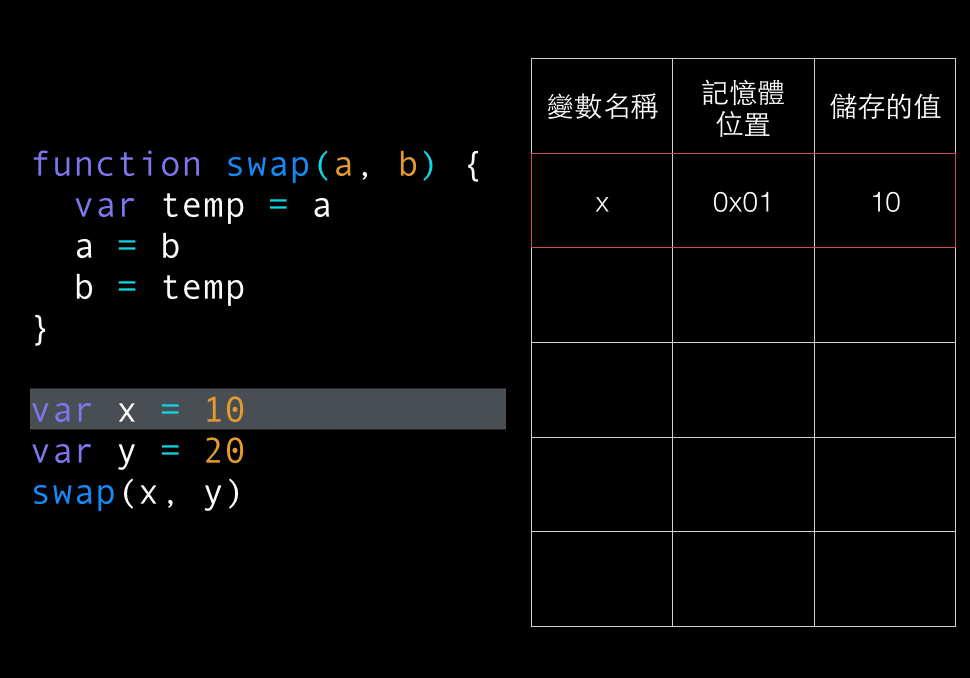```function add(obj) {
obj.number++
}

var o = {number: 10}
console.log(o.number) // 11```

```function add(obj) {
// 讓 obj 變成一個新的 object
obj = {
number: obj.number + 1
}
}

var o = {number: 10}
console.log(o.number) // 10```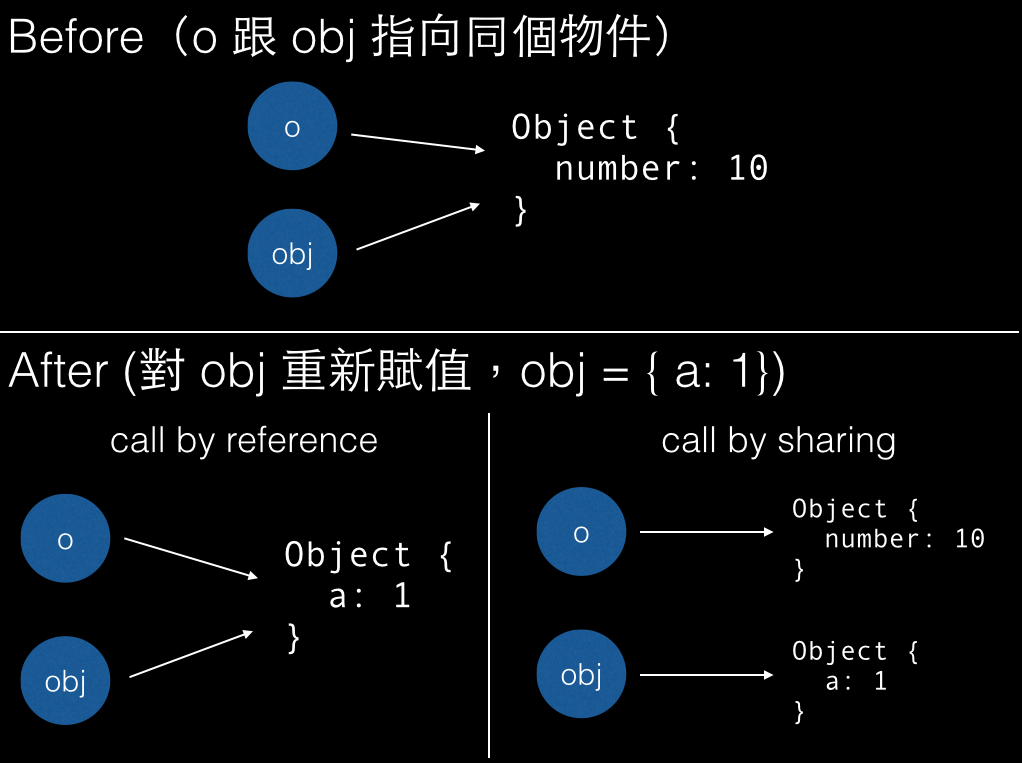# JavaScript 只有 call by value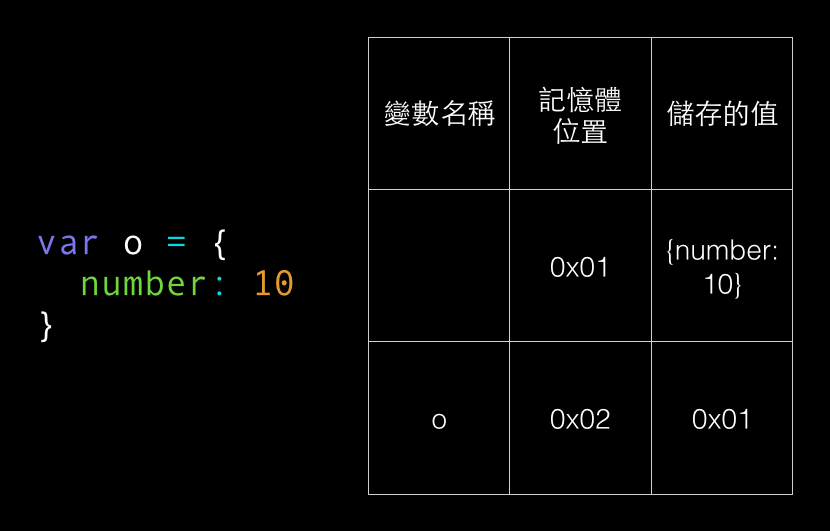o 這個變數的值是什麼？

# 探索聖經的路程

ECMAScript 的 spec 就是 JavaScript 的聖經，在裡面你可以找到更底層的實作，而且內容絕對不會出錯。

It can be either “call by value”, with specifying that the special case of call by value is meant — when the value is the address copy. From this position it is possible to say that everything in ECMAScript are passed by value.

Or, “call by sharing”, which makes this distinction from “by reference”, and “by value”. In this case it is possible to separate passing types: primitive values are passed by value and objects — by sharing.

The statement “objects are passed by reference” formally is not related to ECMAScript and is incorrect.

1. call by reference
2. call by value
3. pass by reference
4. pass by value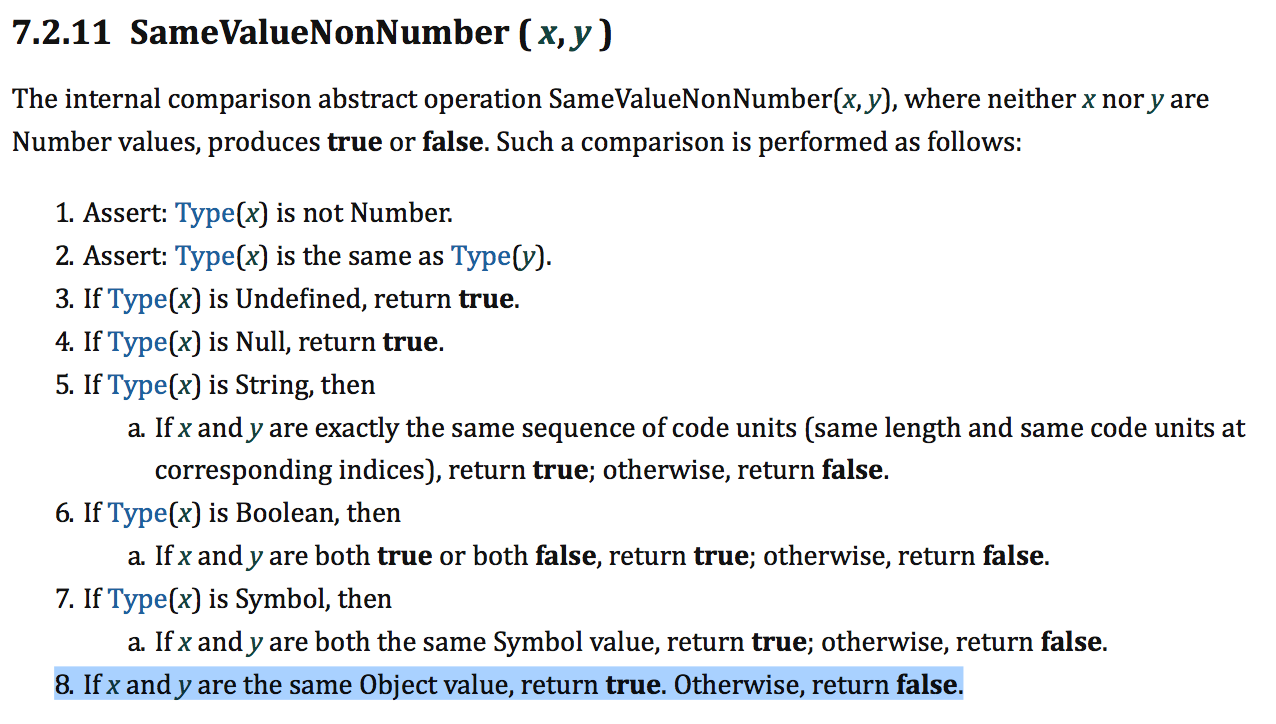1. If x and y are the same Object value, return true. Otherwise, return false.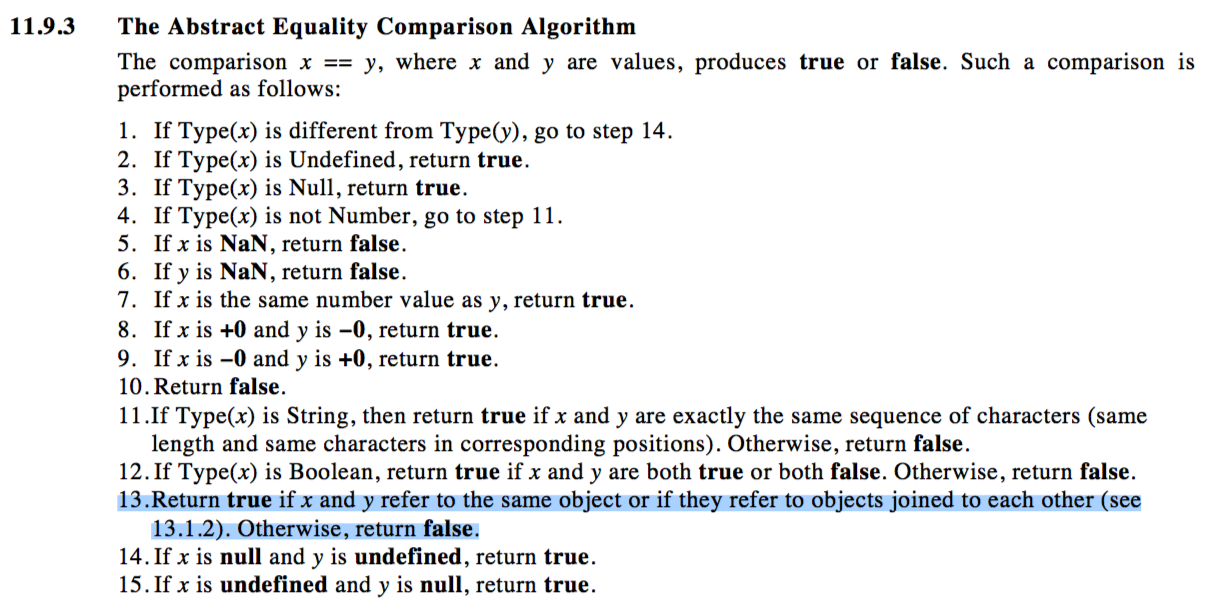11.9.3 The Abstract Equality Comparison Algorithm

13.Return true if x and y refer to the same object or if they refer to objects joined to each other (see 13.1.2). Otherwise, return false.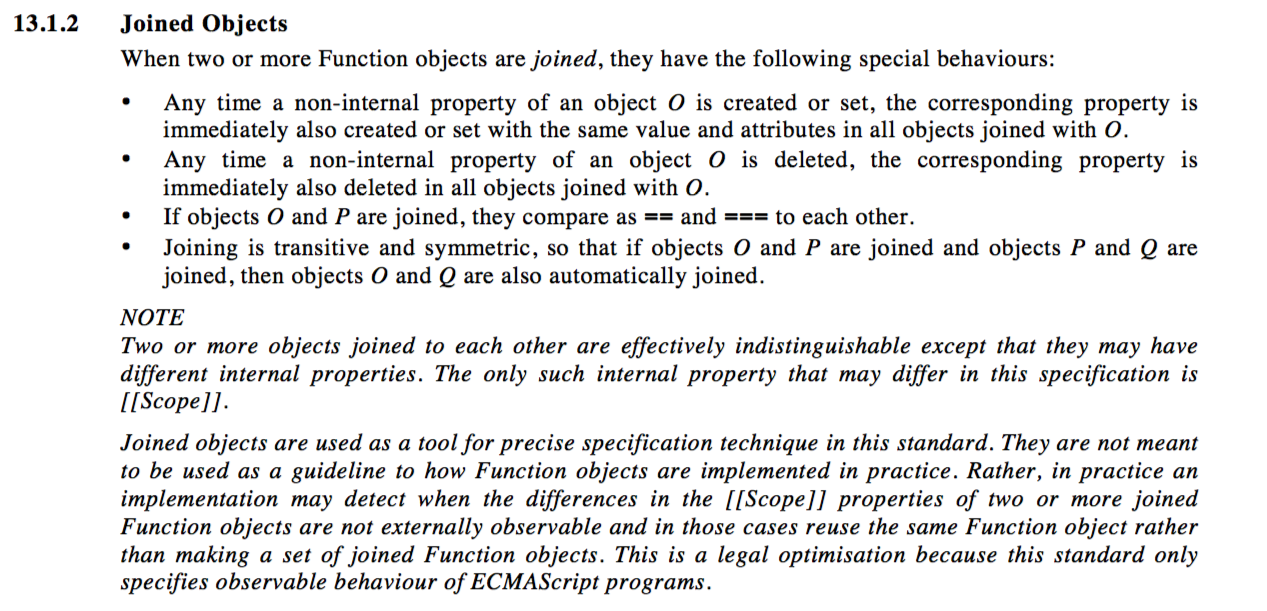# Java is always pass-by-value

However, Objects are not passed by reference. A correct statement would be Object references are passed by value.

Now that we have some definitions of terms we can return to the question. Does Java pass objects by reference or by value?

The answer is NO! The fact is that Java has no facility whatsoever to pass an object to any function! The reason is that Java has no variables that contain objects.

The reason there is so much confusion is people tend to blur the distinction between an object reference variable and an object instance. All object instances in Java are allocated on the heap and can only be accessed through object references. So if I have the following:

StringBuffer g = new StringBuffer( "Hello" );

The variable g does not contain the string "Hello", it contains a reference (or pointer) to an object instance that contains the string "Hello".

g 這個變數的值並不是字串`Hello`，而是`一個指到字串 Hello 的 reference`，所以你在呼叫 function 的時候，傳進去的就是這個 reference。

Java 中 Call by value，指的是傳遞參數時，一律傳遞變數所儲存的值，無論是基本型態或是類別宣告的型態都一樣，Java 中不允許處理記憶體位址，所以用了「參考」這個名稱來作為解釋類別型態所宣告的變數之行為，但這邊的「參考」與 C++ 中所稱之「參考」，是完全不相同的行為，更不會有 C++ 中參數的傳值、傳參考、return 的傳值、傳參考的 Call by reference 行為。

Before we leave the topic of manipulating objects and arrays by reference, we need to clear up a point of nomenclature.

The phrase "pass by reference" can have several meanings. To some readers, the phrase refers to a function invocation technique that allows a function to assign new values to its arguments and to have those modified values visible outside the function.

This is not the way the term is used in this book. Here, we mean simply that a reference to an object or array -- not the object itself -- is passed to a function. A function can use the reference to modify properties of the object or elements of the array. But if the function overwrites the reference with a reference to a new object or array, that modification is not visible outside of the function.

Readers familiar with the other meaning of this term may prefer to say that objects and arrays are passed by value, but the value that is passed is actually a reference rather than the object itself

# C 與 C++ 的參數傳遞

```#include <stdio.h>

void swap(int a, int b) {
int temp = b;
b = a;
a = temp;
}

int main(){
int x = 10;
int y = 20;
swap(x, y);
printf("%d %d\n", x, y); // 10, 20
}```

```#include <stdio.h>

void swap(int *a, int *b) {
// 印出 a 跟 b 所存的值
printf("%ld, %ld", a, b); //0x44, 0x40
int temp = *b;
*b = *a;
*a = temp;
}

int main(){
int x = 10;
int y = 20;
// 印出 x 跟 y 的記憶體位置
printf("%ld %ld\n", &x, &y); // 0x44, 0x40
swap(&x, &y); // 傳記憶體位置進去
printf("%d %d\n", x, y); // 20, 10
}```

```#include <stdio.h>

void swap(int *a, int *b) {

// 印出 a 跟 b 所存的值
printf("%ld, %ld", a, b); //0x44, 0x40
int temp = *b;
*b = *a;
*a = temp;
}

int main(){
int x = 10;
int y = 20;

// 兩個指標指向 x 跟 y
int* ptr_x = &x;
int* ptr_y = &y;

// 印出 x 跟 y 的記憶體位置（就是 ptr_x 跟 ptr_y 存的值）
printf("%ld %ld\n", ptr_x, ptr_y); // 0x44, 0x40
swap(ptr_x, ptr_y); // 傳記憶體位置進去
printf("%d %d\n", x, y); // 20, 10
}```

```#include <stdio.h>

// 注意到這邊多了 &，其他都跟 call by value 一模一樣
void swap(int &a, int &b) {

// 印出 a 跟 b 所存的值與記憶體位置
printf("%ld, %ld\n", a, b); // 10, 20
printf("%ld, %ld\n", &a, &b); // 0x44, 0x40
int temp = b;
b = a;
a = temp;
}

int main(){
int x = 10;
int y = 20;

// 印出 x 跟 y 的記憶體位置
printf("%ld %ld\n", &x, &y); // 0x44, 0x40
swap(x, y); // 傳記憶體位置進去
printf("%d %d\n", x, y); // 20, 10
}```

call by value 會把傳進去的值複製（無論那個值是數字也好，記憶體位置也好，都會複製一份），call by reference 在「最底層的實作」上當然也會有類似的行為，但是你感覺不出來。

# 結論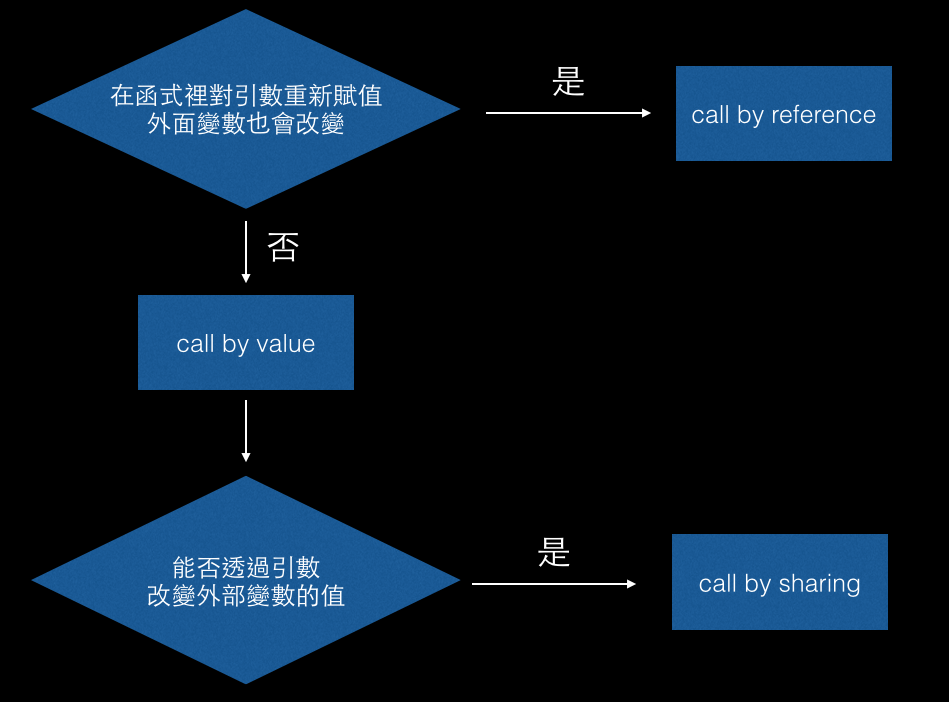1. JavaScript 裡面只有 pass by value
2. JavaScript 的 primitive type 是 pass by value，object 是 pass by sharing

# 參考資料

###MapleShaw commented Feb 21, 2019

 学习了！！！写得太好了！！！

###aszx87410 commented Feb 21, 2019

 @MapleShaw 感謝！
This was referenced Feb 23, 2019

###rockmiao commented Mar 26, 2019

 其實我自己本身的理解是： 在函式外部宣告的obj 其底層有一個pointer指向他真實記憶體位址 當你丟進去某函式時，函式的參數也會去指向同一個記憶體位址 所以你對這個記憶體位址去做改變 外部跟著改變很正常 但是當你重新賦值，就變成是重新建立一個obj 並且讓函式的參數去指向那個新的obj記憶體位址 所以改變不到外部的 不知道這個理解行不行？

###aszx87410 commented Mar 26, 2019

 @rockmiao 差不多是這樣

###aszx87410 commented Dec 17, 2019

 原來在 JS 裡面 arguments 跟 call by reference 的表現一樣 ```function run(a){ arguments = {name: 'run'} console.log(a) } var obj = {name: 'obj'} run(obj)``` 就算重新賦值，一樣會改變原本 a 的值